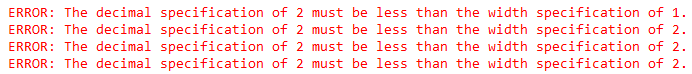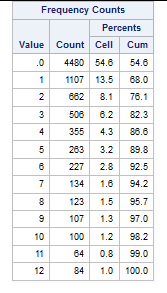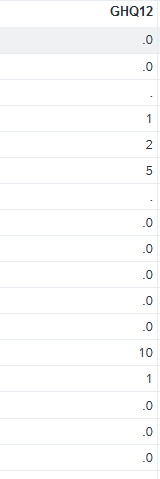## Trichotomization

I have GHQ12 which has 13 different cohorts. I need to make it a variable with only 3 categories.

I used the following code:

``````data work.sampsys1;
set work.sampSys;
If GHQ12 = 0 then GHQ3 = 1;
If GHQ12 > 0 and GHQ12 < 3 THEN GHQ3 = 2;
else GHQ3 = 3;
run;``````

I have the following error:This is the frequency table for GHQ12:6 REPLIES 6

## Re: Trichotomization

I would rewrite the program as follows

``````data work.sampsys1;
set work.sampSys;
If GHQ12 = 0 then GHQ3 = 1;
else If 0 < GHQ12 < 3 then GHQ3 = 2;
else GHQ3 = 3;
run;``````

and that should be programatically correct.

Unless the error is to do with code you haven't provided it shouldn't occur now.

## Re: Trichotomization

there also seems to be a .0 in the frequency table which may need addressing

## Re: Trichotomization

@33pedro wrote:
there also seems to be a .0 in the frequency table which may need addressing

Tried to address it. Not really working. Maybe because I do not know how to address .o in my dataset!

``````data work.impo;
set work.import;
If GHQ12 = .0 then GHQ3 = 1;
else If .0 < GHQ12 < 3 then GHQ3 = 2;
else GHQ3 = 3;
run;``````

Best regards,

## Re: Trichotomization

Thank you so much, Pedro. Still, I have the same error. I checked GHQ12 and this is some units of this variable:I tried your code on the different data set, still, I have the exact same error.

## Re: Trichotomization

I assume from the . values that this is a numeric variable? The .0 values look a bit unusual which is why I am asking.

try this

``````data work.sampsys1;
set work.sampSys;
if GHQ12 = .0 then GHQ12 = 0;
If GHQ12 = 0 then GHQ3 = 1;
else If 0 < GHQ12 < 3 then GHQ3 = 2;
else GHQ3 = 3;
run;``````

That should at least tell us if the .0 is causing the problem

## Re: Trichotomization

Hello @Sahar775 and welcome to the SAS Support Communities!

Most likely, the error messages are caused by invalid formats (e.g. w.d formats such as 1.2 or 2.2) which are permanently assigned to GHQ12 and other variables in dataset WORK.SAMPSYS. (PROC SQL might have assigned these formats.)

To see which variables are affected, run a PROC CONTENTS step like

``````proc contents data=work.sampsys;
run;``````

and look at column "Format."

If all formats there are invalid (such as 2.2) or unnecessary, you can remove all formats with PROC DATASETS:

``````proc datasets lib=work nolist;
modify sampsys;
format _all_;
quit;``````

Otherwise, you can specify individual valid formats and drop others as well by replacing format _all_; with a valid format specification in the above step. (Get back to us if you need help with this.)

Once you have changed/removed the assigned formats, check if the following step creates error messages:

``````data _null_;
set work.sampsys(obs=0);
run;``````

If the log is clean, you're ready to run your DATA step to create WORK.SAMPSYS1.

Discussion stats
• 6 replies
• 537 views
• 2 likes
• 3 in conversation